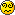## Problem 2: Cow-Bot

The cows sense victory. With the monkeys "pinned" squarely in the middle of Scarberia, attending to their tender behinds, the cows have decided to go in for the kill. Before they do so, however, they must gather some intelligence. To this end, the leader of the cows, Bo Vine, has designed a robot. He calls it… (Insert drum roll here)… COW-BOT! As with every computer program, ahem, Cow-Bot has to be tested rigorously and thoroughly to ensure that there are no flaws in his implementation.

Bo Vine has fed into the robot a list of instructions that it must execute. Cow-Bot will be presented with an initial number and be given the number of iterations it must perform. He begins by considering instructions at the beginning of the list, and executes the first of these that apply. The result of the action is the number used in the following iteration, which again begins at the top of the list.

Condition Action
Number is primeMultiply by 11; e.g.: 2 → 22
Number is a perfect squareAdd the reverse of the number; e.g.: 64 → 110
Number is a palindromeAdd a 4 at the end; e.g.: 11 → 114
Number starts with a 2Add a 5 at the front; e.g.: 21 → 521
Number has a 7 anywhere in itRemove the last digit; e.g.: 27 → 2
Number is divisible by 6Remove the first digit; e.g.: 108 → 8
Number has an even number of digits    Place a 1 in the middle; e.g.: 42 → 412
Number has an odd number of digitsAdd 231; e.g.: 1 → 232

### Input

The first line of input contains a single integer T (1 ≤ T ≤ 50), indicating the number of test cases.
Each test case consists of one line of 2 numbers, a and b, where a (0 ≤ a ≤ 214783647), is the starting number and b (0 ≤ b ≤ 32767), is the number of iterations to be performed.

### Output

For each case, output the number that is the final result of all computations.

```2
5 3
545 5
```

### Sample Output

```785
5144
```

Note: we guarantee that any result, intermediate or final, that is produced by the Cow-Bot is not greater than 2147483647.

Point Value: 10
Time Limit: 2.00s
Memory Limit: 16M

Languages Allowed:
C++03, PAS, C, HASK, ASM, RUBY, PYTH2, JAVA, PHP, SCM, CAML, PERL, C#, C++11, PYTH3

• (2/0)
If the number is divisible by 6 the first digit is deleted. Assuming the number at this point is 6 itself, there would be no number left. What happens at this point?• (2/0)
Assume that it becomes a zero.

• (0/0)
Compiled and ran fine with test data on my home computer. Gives WA here.

I know there are some differences between GCC and MinGW (such as 'count' being a reserved word in GCC) but I can't seem to find any in my program. Does anyone know what it might be?

• (0/0)
It is not a compiler issue.
The problem lies in your code.

• (0/0)
Only it works fine on MinGW, WinXP 32bit.
I tried it with Judge test data from my Pascal account...

• (0/0)
Why am I getting runtime error? I compiled it and ran it on my computer and it worked fine.

• (0/0)
Look at the error - it's SIGFPE. If you look in the help section, you'll find that it means you're improperly using a math function... there's only one possibility in your code. It should be fairly clear why it's giving you an error.